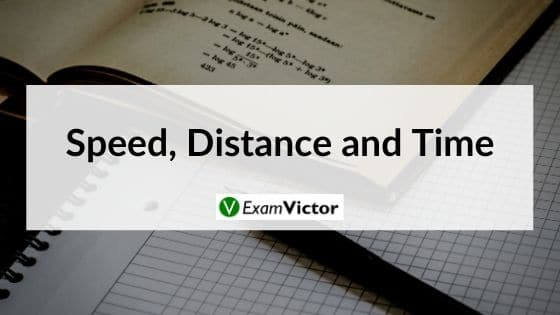# Speed, Distance and TimeLast Updated on Nov 11, 2020

##### Average Speed Formula

Average speed = Total Distance covered/ Total Time Taken

When the distance is constant: Average speed = 2xy/x+y; Where, x and y are the two speeds at which the same distance has been covered.

When time taken is constant: Average speed = (x + y)/2; Where, x and y are the two speeds at which we traveled for the same time.

##### Time and Distance Formula

Distance = Speed × Time.

Using this formula, all basic problems can be handled. However, you need to make sure about the correct usage of units while using the above formulas.

Speed is inversely proportional to the time taken when the distance traveled is constant. So, when speed increases, time decreases and vice versa.

Relative Speed:
Relative speed is defined as the speed of a moving object with respect to another. When two objects are moving in the same direction, the relative speed is calculated as their difference. When the two objects are moving in opposite directions, the relative speed is computed by adding the two speeds.

Example: A person goes from A to B at the speed of 40 kmph and comes back at the speed of 60 kmph. What is his average speed for the whole journey?

Solution: Since the distance traveled on both sides is the same, we can use the formula of harmonic mean of speeds.

Average Speed: 2xy/(x+y) where, x is the speed while going from A to B and y is the speed while coming back.
So using this formula, we get the answer as 48 kmph.

Example: Moving at 50 kmph, a person reaches his office 10 min late. Next day, he increases his speed and moves at 60 kmph and reaches his office 5 min early. What is the distance from his home to his office?

Solution: We can observe that difference in timings on both days is 15 min (and not 5 min, as one day he is late and on the other day he is early)

Let the required distance = D km. As time taken at the speed of 50 kmph is more than time taken at 60 kmph, so equation can be formed as D/50-D/60=15/60. Solving this equation, we get the answer as 75 km.

Always remember that during solving questions units must be same. Units can be km/hr, m/sec etc.

Conversion of km/ hr to m/ sec and m/ sec to km/ hr

x km/ hr = (x* 5/18) m/sec i.e. u just need to multiply 5/18

Similarly, x m/sec = (x*18/5) km/sec

Example: A man covers a distance of 600m in 2min 30sec. What will be the speed in km/hr?

Solution: Speed =Distance / Time
⇒ Distance covered = 600m, Time taken = 2min 30sec = 150sec
Therefore, Speed= 600 / 150 = 4 m/sec
⇒ 4m/sec = (4*18/5) km/hr = 14.4 km/ hr.

Example: A car travels along four sides of a square at speeds of 200, 400, 600 and 800 km/hr. Find average speed.

Solution: Let x km be the side of the square and y km/hr be the average speed
Using the basic formula,
Time = Total Distance / Average Speed

x/200 + x/400 + x/600 + x/800 = 4x/y ⇒ 25x/ 2400 = 4x/ y⇒ y= 384
⇒ Average speed = 384 km/hr

This video lays out the basics of Speed, Distance and Time. As you may know, this topic is an exam-setter's favorite. Understanding this topic well is necessary to solve questions that are asked in various competitive exams in the Logical Reasoning and Quant section - applicable to CAT, XAT, MAT, SNAP, IIFT, CLAT, AILET, DU LLB, any other entrance exam as well.